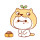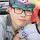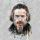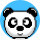# WordPress淘宝客插件 (一键获取及自动填充商品信息和推广链接)

★查看WordPress淘宝客插件/主题各版本功能及价格对比

★购买WordPress淘宝客APP/小程序

QQ咨询: 3249892、QQ群讨论：77434617

## 功能

1.兼容所有的主题，也就是您不用换主题也能使用本插件。

*本身有商品模块的主题建议您【对接主题】

(1). 支持将普通主题【改造成淘宝客主题】，自动在文章中插入【去购买】链接或者显示丰富的【商品模版】，看演示

(2). 使用编辑器【淘】插入商品，一篇文章一个商品的不推荐使用此方式，建议用【改造成淘宝客主题】，因为【淘】插入的目前不支持自动更新商品优惠信息等（可以看后面的第9点）。

(3). 支持 WooCommerce 一键对接，只需要在【对接主题】开启即可。（点击看图，当前仅限旗舰版及以上版本）

(4). 如果您正在使用本站开发的WordPress淘宝客主题，现在找到更好的模版了，想替换本站主题，又要兼容旧商品数据，建议购买淘宝客插件旗舰版/尊享版，完美兼容新旧数据。

——如果您的新主题是个淘宝客相关的主题，那么你只需要在【对接主题】配置想要的自定义字段即可。
——如果您的新主题是普通的文章类主题，那么你只需要在【对接主题】开启【我用的是普通主题】和【改造成淘宝客主题】-“文章页”选择一种，考虑勾选使用【商品默认模版】。
——如果您打算或者已经对我们的官方淘宝客主题二次开发过（魔改），即商品数据依然保存到自定义字段 tbk 中，建议使用淘宝客插件吧，你只需要在【对接主题】开启【我用的是普通主题】即可，你想要的手动发布，软件采集、自动采集等等都兼容。

2. 一键获取商品信息，发布商品效率高，小编最爱。

（1）在不改变您原有主题的结构下，增加一个获取信息栏目，只要您在商品链接那边输入一个商品链接或者推广链接，点击`【获取信息】`就可以拿到标题，图标、价格、优惠券链接等信息。①. 提示商品通用佣金多少，月支出佣金及推广量，可以初步判断商品受欢迎程度。（目前仅限淘宝/天猫/京东/拼多多/考拉海购）
②. 推广链接会自动填充，支持高佣金（仅限淘宝/天猫）。

`补充说明：`

①. 获取商品信息目前支持淘宝网/天猫/京东/拼多多/苏宁/考拉海购/国美/当当网/聚划算等。（包括获取推广链接）
②. 支持 多麦CPS广告联盟（仅获取推广链接） ，可以一次申请上百个商城(如:京东/国美/苏宁/当当网/唯品会/一号店等)，申请快速，收款更快!
③. 支持自动获取亚马逊中国/美国亚马逊推广链接。

（2）在文章中插入多个商品
①. 在后台发布页面，点击可视化编辑器【淘】按钮，支持任意商品（点击看图），且提供多套优美模版。### 一片式无痕无钢圈文胸聚拢调整型小胸罩厚薄款少女士隐形性感内衣

②. 支持将文章中的淘宝、天猫、京东商品链接自动转换为推广链接，淘宝、天猫优惠券链接也支持自动转换为推广链接，并且链接对外显示为`内链`。（只是替换链接，保持原来模版风格，该功能为旗舰版及以上版本独有。

（3）调用其他文章的商品

3.智能申请高佣计划（含营销计划）

4. 微信公众号找券

5. 支持自动采集商品和使用其他软件采集商品

（1）. 全自动采集商品 —— 不用购买付费采集软件、不用24小时挂机采集、不用写烦人的采集规则。

PS:目前仅支持淘宝、天猫、京东、拼多多。

（2）. 使用其他软件采集商品，需要自己准备采集工具，比如火车头、简数采集平台，需要您自己解决采集规则，我们会自动把别人的淘宝客链接转为您的。

PS:采集时如果包括优惠券，会自动返回优惠券面值/截止日期等。

PS:目前不支持转换文章正文中出现的推广链接。仅适合采集单个直达链接。

6. 管理员可以给小编分配PID，方便小编或者合伙人参与分成或者考核小编业绩。

7.替换主题自带的内链方式，让主题与时俱进，比如自动拼接单品+优惠券二合一，微信APP/微博APP中显示淘口令等。

（1）内链格式 `http://您的网址/go/xxx`
（2）好处：

②. 可以分享内链到朋友圈、QQ、QQ群、微博等，不会违背淘宝联盟规则，否则很容易因为渠道不对被扣分。

8. 淘口令

9.自动检查商品是否下架，优惠券是否更新、过期或者领完。

10.如果您网站修改域名或者修改PID，前台点击购买后会自动帮你改为最新的PID。（需要开启内链）

## 设置插件

#### 跟您的主题对接PS:如果没有看到自定义栏目，请看编辑文章页面右上角，看到有一个【显示选项】，点击它，然后勾选【自定义栏目】#### (2).或者节点ID方式对接※ 获取节点ID：、或者 右键-查看网页源代码：※ 如果没有节点ID，也可以使用name值：

`post-body textarea[name=buylink_value]`

`post-body input[name=buylink_value]`

## 联盟设置

### 1.淘宝联盟

PS: PID/找券/微信找券/选品库/定向招商等pid建议不要使用同一个，方便查看报表【效果报表-自助推广】

### 2.多麦CPS广告联盟PS:如果您使用多麦CPS广告联盟，京东/国美/苏宁/当当网联盟也可以直接使用多麦的。

### 7.当当网联盟### 9.亚马逊网站联盟PS:如果您使用多麦CPS广告联盟，京东/国美/苏宁/当当网联盟也可以直接使用多麦的。

## 支持将别人的推广链接转为自己的

```s.click.taobao.com
g.click.taobao.com
i.click.taobao.com
redirect.simba.taobao.com
uland.taobao.com/coupon/edetail
u.jd.com
union-click.jd.com
union.click.jd.com
sucs.suning.com/visitor.htm
union.dangdang.com/transfer.php
www.amazon.cn
c.duomai.com/track.php
p.yiqifa.com
```

## 常见问题

1、对服务器有什么要求?

PHP版本不限制（支持PHP 5.2~PHP 8）

2、启用插件时出现如下错误： ..xxx.php:3

3、是一次性收费还是每年收费？我能免费升级吗?

4、我能退货吗?

5、我用了一段时间后，能更换域名吗？

6、提供技术支持吗?

7、我应该选择单域名/根域名/WPMU中的哪一类型?

8、安装时应该注意什么？

## 更新日志

```= 4.5.8 =

2021/09/26

【自动采集】新增过滤规则：只采集包含商品详情的商品（仅淘宝/天猫）

= 4.5.7 =

2021/07/31

【自动采集】支持选择使用短标题或长标题
【自动采集】支持选择是否采集文章标签

= 4.5.6 =

2021/07/16

= 4.5.3 =

2021/05/07

【改造成淘宝客主题】新增简码，可以在文章中任意位置插入及显示商品模块。

= 4.5.2 =

2021/01/19

【改造成淘宝客主题】移到【对接主题】，新增支持使用【商品默认模版】，与【淘】插入的模版相同，不开启则仅显示【去购买】链接。

= 4.5 =

2021/01/08

= 4.4 =

2020/12/31

= 4.3.3 =

2020/10/27

= 4.3.2 =

2020/10/21

= 4.3.1 =

2020/10/18

= 4.3 =

2020/9/30

【自动采集】新增拼多多

= 4.2 =

2020/4/25

= 4.1 =

2019/12/30

= 4.0 =

2019/9/27

【自动采集】

= 3.7.3 =

2019/7/1

= 3.7.2 =

2019/5/13

= 3.7.1 =

2019/4/6

= 3.7 =

2019/2/2

= 3.6.9 =

2019/1/11

= 3.6.8 =

2019/1/5

= 3.6.7 =

2018/12/29

= 3.6.5 =

2018/12/14

= 3.6.4 =

2018/11/28

= 3.6.3 =

2018/11/6

【广告窗】新增：网站最顶部的广告条

= 3.6.2 =

2018/10/23

【自动采集】规则新增过滤标题，支持黑/白名单。

= 3.6.1 =

2018/8/31

= 3.6 =

2018/8/24

= 3.5.6 =

2018/7/18

= 3.5.5 =

2018/7/13

= 3.5.4 =

2018/6/26

= 3.5.3 =

2018/5/14

= 3.5.2 =

2018/4/24

= 3.5 =

2018/3/7

= 3.4 =

2017/11/1

= 3.3.6 =

2017/10/20

= 3.3.5 =

2017/9/5

= 3.3.3 =

2017/8/31

= 3.3.2 =

2017/7/26

= 3.3 =

2017/7/22

= 3.2.4 =

2017/1/5

= 3.2.3 =

2017/1/1

= 3.2.2 =

2016/12/21

= 2.4.1 / 3.2.1 =

2016/12/16

= 2.4 / 3.2 =

2016/12/14

= 3.1 =

2016/11/30

= 3.0.4 =

2016/11/29

= 3.0 =

2016/11/28

= 2.2.1 =

2016/11/21

= 2.1 =

2016/9/28

= 2.0 =

2016/9/17

= 1.6.4 =

2016/8/6

= 1.6.3 =

2016/6/4

= 1.6.2 =

2016/4/12

= 1.6.1 =

2016/3/23

【淘】插入的商品去掉淘宝客链接判断，支持使用各种短网址了。（无需修改以前的商品）
【商品链接】支持这类短网址：http://t.cn/xxxxxx http://dwz.cn/xxxxxx

= 1.6 =

2016/3/21

【获取信息】支持直接转换他人的推广链接了，目前支持淘宝、京东、苏宁、当当网、多麦、亚马逊、亿起发等的推广链接。

= 1.5.3 =

2016/3/19

= 1.5.2 =

2015/12/4

= 1.5.1 =

2015/12/3

= 1.5 =

2015/12/2

= 1.4.9 =

2015/12/1

= 1.4.8 =

2015/11/28

= 1.4.7 =

2015/11/27

= 1.4.6 =

2015/11/19

= 1.4.4 =

2015/11/15

= 1.4.2 =

2015/11/14

= 1.4 =

2015/11/13

= 1.3.1 =

2015/08/08

= 1.3 =

2015/08/02

= 1.2.4 =

2015/07/31

= 1.2.3 =

2015/07/30

= 1.2 =

2015/07/23

= 1.1.1 =

2015/07/21

= 1.1 =

2015/07/20

= 1.0 =

2015/07/17

```1.梁宝宝

什么时候 “支持将别人的推广链接转为自己的” 支持A链接啊

2021年5月20日 21:08
2.David.Lee

怎么把商品添加到WooCommerce？

2019年12月9日 14:28
3. 好像对php7.2支持不理想，会报错

2018年11月9日 00:12来自iPhone
• 这是服务器配置问题，因为7.2好像默认开启了各种通知，如果是宝塔面板，可以【点击这里】看截图设置

2018年11月10日 20:27
4.張志任

2018年11月7日 11:08来自QQ
5.肉球

我用了测试版，发现插入文章中的那个商品有太正式化了，如果可以自定义图片大小就好了，比如说一个图片是1000*700的，不想这么明目张胆的插入商品。跟你的前台样式的第三个一样，但是图片是自适应的就更好了。

2018年7月9日 20:54
6.kaho100

8.